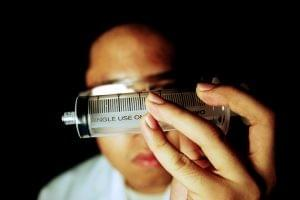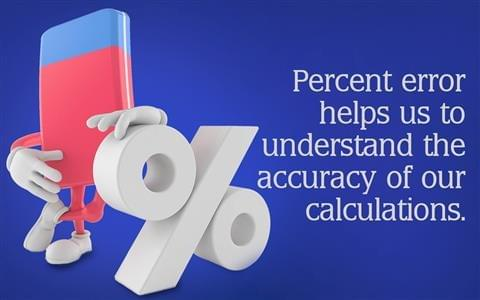• # Calculate percent error

#### DefinitionPercent error or percentage error expresses as a percentage the difference between an approximate or measured value and an exact or known value. It is used in science to report the difference between a measured or experimental value and a true or exact value. Here is how to calculate percent error, with an example calculation.### Percentage error terminology

When conducting an experiment the theoretical value is often referred to as the true value. This is the value expected to be seen when conducting a measurement. The observed value can also be called the experiment value and is the actually measured when conducting a measurement. Now you can easily find percent error using the formula or our handy calculator. Use our percentage calculators for more tools for calculating percent values.

Key Points: Percent Error
• The purpose of a percent error calculation is to gauge how close a measured value is to a true value.
• Percent error (percentage error) is the difference between an experimental and theoretical value, divided by the theoretical value, multiplied by 100 to give a percent.
• In some fields, percent error is always expressed as a positive number. In others, it is correct to have either a positive or negative value. The sign may be kept to determine whether recorded values consistently fall above or below expected values.
• Percent error is one type of error calculation. Absolute and relative error are two other common calculations. Percent error is part of a comprehensive error analysis.
• The keys to reporting percent error correctly are to know whether or not to drop the sign (positive or negative) on the calculation and to report the value using the correct number of significant figures.### Percent error calculation steps

1. Subtract one value from another. The order does not matter if you are dropping the sign, but you subtract the theoretical value from the experimental value if you are keeping negative signs. This value is your "error."

2. Divide the error by the exact or ideal value (not your experimental or measured value). This will yield a decimal number.

3. Convert the decimal number into a percentage by multiplying it by 100.

4. Add a percent or % symbol to report your percent error value.